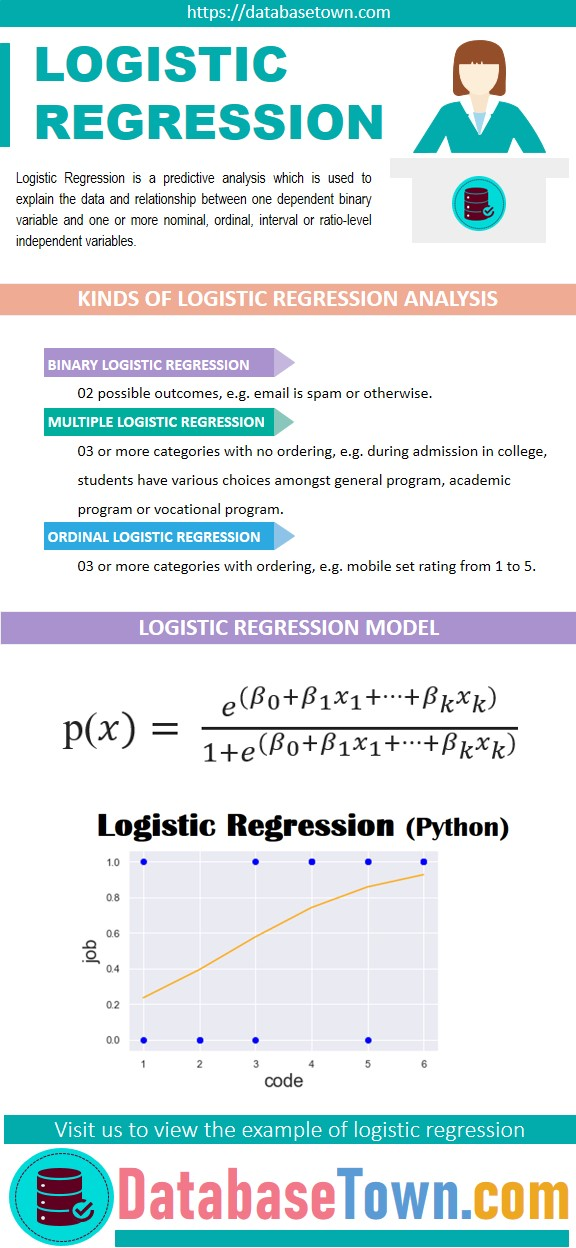# Logistic Regression (Python) Explained using Practical Example

Logistic Regression is a predictive analysis which is used to explain the data and relationship between one dependent binary variable and one or more nominal, ordinal, interval or ratio-level independent variables. It is mostly used in biological sciences and social science applications. For instance, predict whether received email is spam or not. Similarly, predict whether customer will purchase the product or not.

Statistical gadgets are used to conduct the analysis as logistic regression is bit difficult to interpret as compare to the linear regression.

There are quite a few kinds of logistic regression analysis are:

1. Binary Logistic Regression – 02 possible outcomes, e.g. email is spam or otherwise.
2. Multiple Logistic Regression – 03 or more categories with no ordering, e.g. during admission in college, students have various choices among general program, academic program or vocational program.
3. Ordinal Logistic Regression – 03 or more categories with ordering, e.g. mobile set rating from 1 to 5.

Logistic Regression Model

## Practical example of Logistic Regression

Import the relevant libraries and load the data.

For quantitative analysis, we must convert ‘yes’ and ‘no’ entries into ‘0’ and ‘1’ as shown in figure.

Now we are going to visualize our data, we are predicting job. Therefore, the job is our Y variable and Code (use for education) will be our X variable.

Here, we observed that for all the observations below the outcomes is zero or they are jobless, whereas, for all the persons above the process are successfully got the job. Now we are going to plot a regression line as shown in below figure.

Linear regression is awesome technique but here it is not suitable for this kind of analysis as this regression does not know that our values are bounded between 0  and 1. Our data is non-linear, therefore, we must have to use non-linear approach. Hence, now we are going to plot a logistic regression curve.

This function depicts the probability of getting job, given an educational code. When the education is low, the probability of getting job is 0 or nill, whereas, the education is high, the probability of getting job is 1 or 100%.

It is clear from the above snap that, when the education is ‘BA’ the probability of getting job is about 60%.

Logistic Regression Summary is shown in below figure.

MLE is stands for Maximum likelihood estimation.

Likelihood function

It is a function that guess how likely it is that the model at hand defines the real fundamental relationship of the variables. Larger the likelihood function, larger the probability that our model is precise.

Maximum likelihood function tries to maximize the likelihood function. Computer going through various values till finds an appropriate model for which the likelihood is the optimum. When there is no more improvement is possible, it will just stop the optimization.

Pseudo R-squared (Pseudo R-squ) is mostly useful for comparing variation of the same model. Different models have the different pseudo R-squares. If the value of Pseudo R-square lies between 0.2 and 0.4, it is considered decent.

LL-Null is stands for Log Likelihood-null. The LL (log-likelihood) of a model which has no independent variables.

LLR is stands for Log Likelihood Ratio which measures if our model is statistically different from LL-Null.

Calculating the accuracy of the model

In order to find the accuracy of the model, we use the results_log.predict() command that return the value predicted by our model. Also apply some formatting to see the results more readable by using this command

np.set_printoptions(formatter={‘float’: lambda x: “{0:0.2f}”.format(x)})

Here, value less than 0.5 means chances of getting jobs is below 50% and the value 0.93 means the chances of getting job is 93%.

Now, we compare the actual value of the model with predicted value

If 90% of the predicted values of the model match with the actual values of the model, we say that the model has 90% accuracy.

In order to compare the predicted and actual values in form of table we use the results_log.pred_table() command as shown in figure.

This result is bit difficult to understand, so we take these results in form of confusion matrix, as shown in below figure

Let’s clear this confusion matrix, for 3 observations the model predicted 0 and the actual vale was also 0, similarly, for 9 observations the model predicted 1 and the actual value was also 1, therefore, the model did its good job here.

Furthermore, for 2 observations the model predicted 0 whereas, the actual value was 1, similarly, 1 observation the model predicted 1 and the actual value was 0, therefore, here the model got confused.

Finally, it depicts from these confusion matrix, the model made an accurate estimation in 12 out of 15 cases which means our model works with (12/15)*100 = 80% accuracy.

We can also calculate the accuracy of the model by using this code

```cm = np.array(cm_df)
accuracy_model = (cm[0,0]+cm[1,1])/cm.sum()*100
accuracy_model```logistic regression python explained